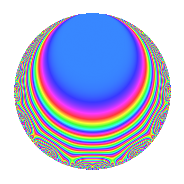# Properties

 Label 2015.1.bfLevel $2015$ Weight $1$ Character orbit 2015.bf Rep. character $\chi_{2015}(309,\cdot)$ Character field $\Q(\zeta_{6})$ Dimension $8$ Newform subspaces $1$ Sturm bound $224$ Trace bound $0$

# Related objects

## Defining parameters

 Level: $$N$$ $$=$$ $$2015 = 5 \cdot 13 \cdot 31$$ Weight: $$k$$ $$=$$ $$1$$ Character orbit: $$[\chi]$$ $$=$$ 2015.bf (of order $$6$$ and degree $$2$$) Character conductor: $$\operatorname{cond}(\chi)$$ $$=$$ $$2015$$ Character field: $$\Q(\zeta_{6})$$ Newform subspaces: $$1$$ Sturm bound: $$224$$ Trace bound: $$0$$

## Dimensions

The following table gives the dimensions of various subspaces of $$M_{1}(2015, [\chi])$$.

Total New Old
Modular forms 16 16 0
Cusp forms 8 8 0
Eisenstein series 8 8 0

The following table gives the dimensions of subspaces with specified projective image type.

$$D_n$$ $$A_4$$ $$S_4$$ $$A_5$$
Dimension 8 0 0 0

## Trace form

 $$8q + 4q^{4} - 4q^{9} + O(q^{10})$$ $$8q + 4q^{4} - 4q^{9} - 4q^{16} - 8q^{25} + 4q^{36} + 8q^{39} + 12q^{41} + 4q^{49} - 8q^{51} - 12q^{59} - 8q^{64} - 4q^{69} + 4q^{81} - 4q^{95} + O(q^{100})$$

## Decomposition of $$S_{1}^{\mathrm{new}}(2015, [\chi])$$ into newform subspaces

Label Dim. $$A$$ Field Image CM RM Traces $q$-expansion
$$a_2$$ $$a_3$$ $$a_5$$ $$a_7$$
2015.1.bf.a $$8$$ $$1.006$$ $$\Q(\zeta_{24})$$ $$D_{12}$$ $$\Q(\sqrt{-155})$$ None $$0$$ $$0$$ $$0$$ $$0$$ $$q+(\zeta_{24}^{5}+\zeta_{24}^{11})q^{3}+\zeta_{24}^{4}q^{4}-\zeta_{24}^{6}q^{5}+\cdots$$